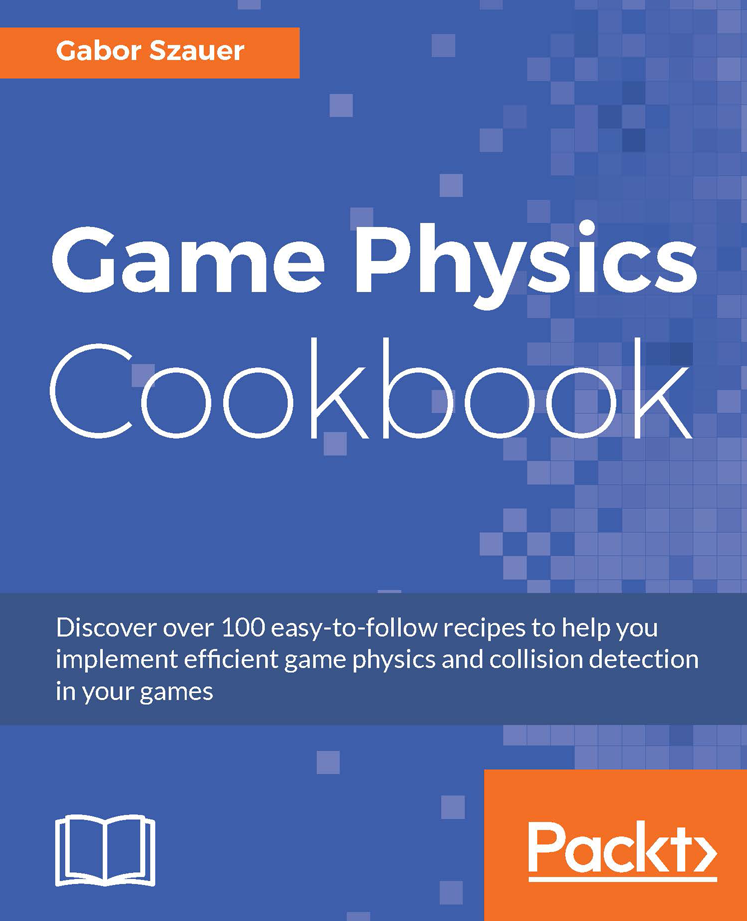# Game Physics Cookbook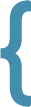# Book overview

This book is a comprehensive guide to the linear algebra and collision detection games commonly use, but only briefly touches on the topic of collision resolution (Physics). The book is structured as follows:

• Chapters 1, 2 and 3 cover the basics Linear Algebra.
• Chapters 4, 5 and 6 cover two dimensional primitives and how to detect intersections between them.
• Chapters 7, 8, 9 and 10 cover three dimensional primitives and how to determine intersections between them.
• Chapters 11, 12 and 13 cover meshes, scenes and scene organization.
• Chapters 14, 15 and 16 cover physics. Throughout these chapters we built a very basic rigid body physics engine.

# Shape collisions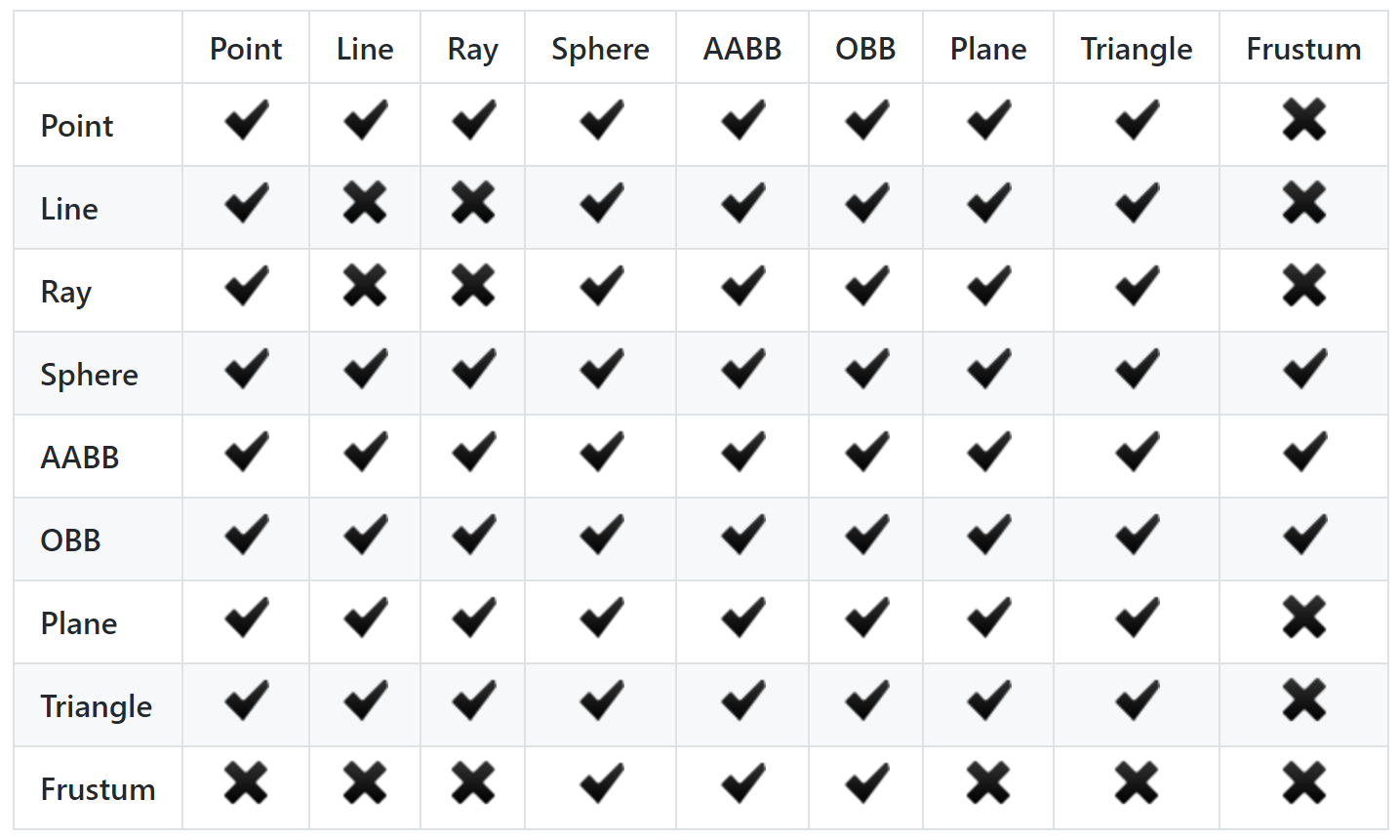# Physics

Chapter 14 covers naive particle physics, this chapter is meant as an introduction to setting up a physics loop and considering the general format of a physics loop. Chapter 15 is the most interesting chapter, it implements a basic rigid body physics engine. The basic engine has support for oriented boxes and spheres, stacking can be made to work but is not directly supported. Chapter 16 covers springs and how springs can be used to implement soft body physics. The final demo of the book is a soft body, cloth physics demo.

##### Chapter 16, Cloth Physics Demo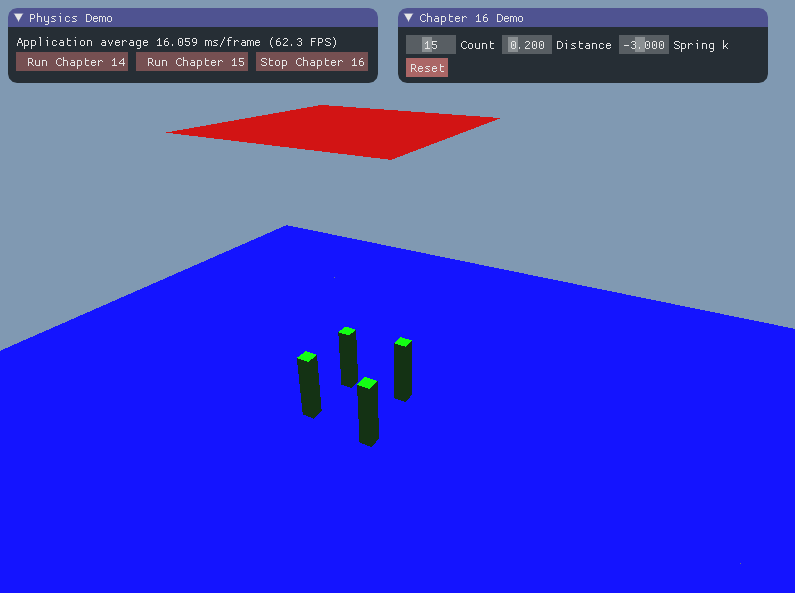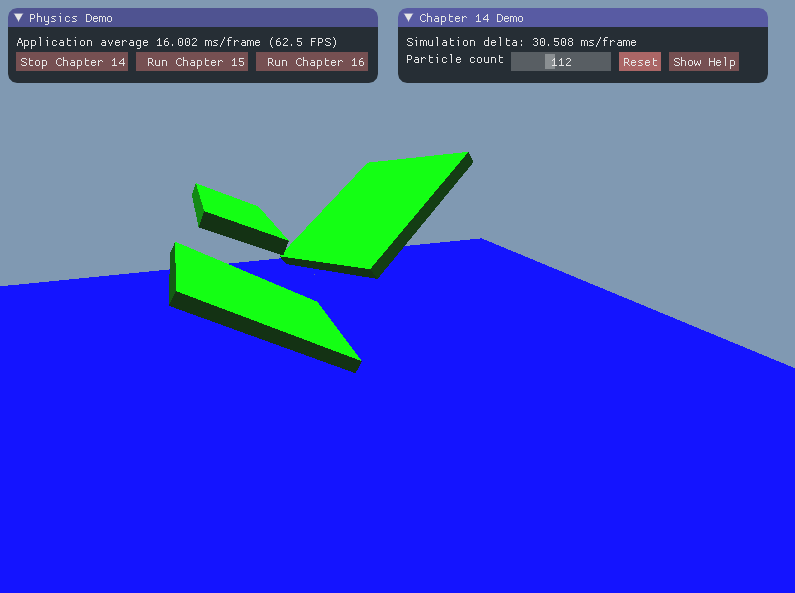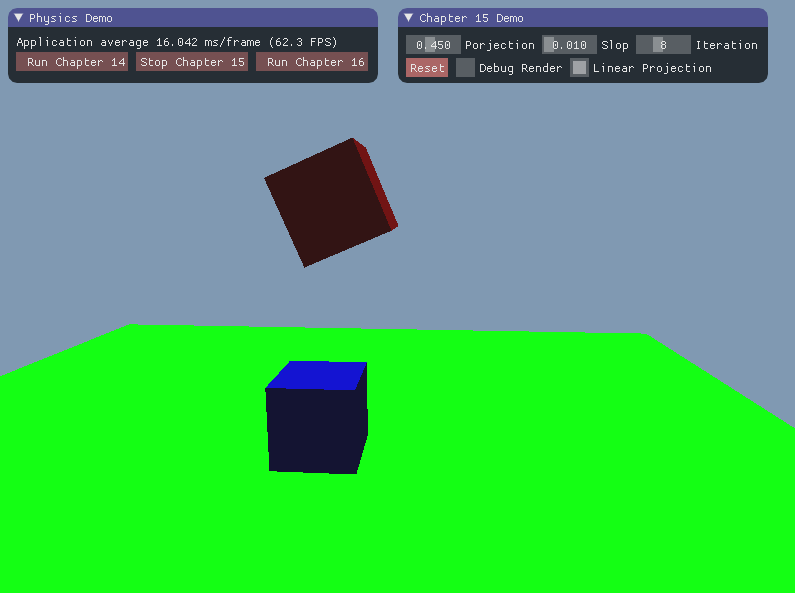Gabor Szauer graduated from Full Sail University with a bachelor's degree in game development. He has been making video games professionally since 2010. He has worked on games for the Nintendo 3DS, Xbox 360, browser-based games as well as games for iOS and Android.

http://gaborszauer.com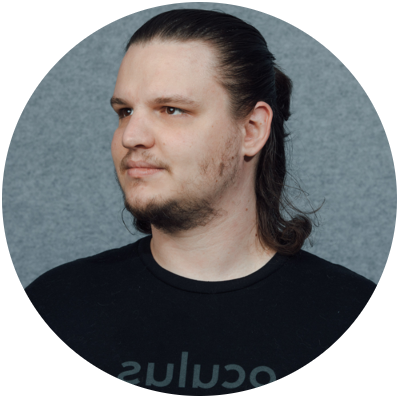1. Vectors
• Introduction
• Vector definition
• Component wise operations
• Dot product
• Magnitude
• Normalizing
• Cross product
• Angles
• Projection
• Reflection
2. Matrices
• Introduction
• Matrix definition
• Transpose
• Multiplication
• Identity matrix
• Determinant of a 2x2 matrix
• Matrix of minors
• Cofactor
• Determinant of a 3x3 matrix
• Operations on a 4x4 matrix
• Matrix inverse
3. Matrix Transforms
• Introduction
• Matrix majors
• Translation
• Scaling
• How rotations work
• Rotation matrices
• Axis angle rotation
• Vector matrix multiplication
• Transform matrix
• Projection matrix
4. 2D Primitive Shapes
• Introduction
• 2D points
• 2D lines
• Circle
• Rectangle
• Oriented rectangle
• Point containment
• Line intersection
5. 2D Collisions
• Introduction
• Circle to circle
• Circle to rectangle
• Circle to oriented rectangle
• Rectangle to rectangle
• Seperating Axis Theorem
• Rectangle to oriented rectangle
• Oriented rectangle to oriented rectangle
6. 2D Optimizations
• Introduction
• Containing circle
• Containing rectangle
• Simple and complex shapes
7. 3D Primitive Shapes
• Introduction
• Point
• Line segment
• Ray
• Sphere
• Axis aligned bounding box
• Oriented buonding box
• Plane
• Triangle
8. 3D Point Tests
• Introduction
• Point and sphere
• Point and axis aligned bounding box
• Point and oriented bounding box
• Point and plane
• Point and line
• Point and ray
9. 3D Shape Intersections
• Introduction
• Sphere to sphere
• Sphere to axis aligned bounding box
• Sphere to oriented bounding box
• Sphere to plane
• Axis aligned bounding box to axis aligned bounding box
• Axis aligned bounding box to oriented bounding box
• Axis aligned bounding box to plane
• Oriented bounding box to oriented bounding box
• Oriented bounding box to plane
• Plane to plane
10. 3D Line Intersections
• Introduction
• Raycast sphere
• Raycast axis aligned bounding box
• Raycast oriented bounding box
• Raycast plane
• Linetest sphere
• Linetest axis aligned bounding box
• Linetest oriented bounding box
• Linetest plane
11. Triangles and Meshes
• Introduction
• Point in triangle
• Closest point triangle
• Triangle to sphere
• Triangle to axis aligned bounding box
• Triangle to oriented box
• Triangle to plane
• Triangle to triangle
• robustness of the seperating axis theorem
• Raycast triangle
• Linetest triangle
• Mesh object
• Mesh optimization
• Mesh operations
12. Models and Scenes
• Introduction
• The model object
• Operations on models
• The scene object
• Operations on the scene
• The octree object
• Operations on the octree
• Octree and scene integration
13. Camera and Frustum
• Introduction
• Camera object
• Camera controls
• Frustum object
• Frusum from matrix
• Sphere in frustum
• Bounding box in frustum
• Octree culling
• Picking
14. Constraint Solving
• Introduction
• Framework introduction
• Raycast sphere
• Raycast bounding box
• Raycast plane and triangle
• Physics system
• Integrating particles
• Solving constraints
• Verlet integration
15. Manifolds and Impulses
• Introduction
• Manifold for spheres
• Manifold for boxes
• Rigid body modifications
• Linera velocity
• Linear impulse
• Physics system update
• Angular velocity
• Angular impulse
16. Sprints and Joints
• Introductions
• Particle modifications
• Springs
• Cloth
• Physics System modification
• Joints